Huffman Encoding Entropy n n Entropy is a

• Slides: 17Huffman Encoding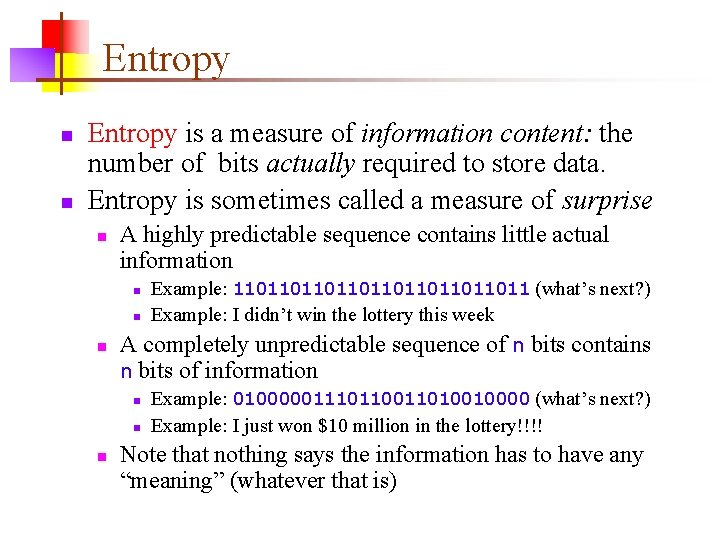Entropy n n Entropy is a measure of information content: the number of bits actually required to store data. Entropy is sometimes called a measure of surprise n A highly predictable sequence contains little actual information n A completely unpredictable sequence of n bits contains n bits of information n Example: 11011011011011 (what’s next? ) Example: I didn’t win the lottery this week Example: 0100000111011010010000 (what’s next? ) Example: I just won \$10 million in the lottery!!!! Note that nothing says the information has to have any “meaning” (whatever that is)Actual information content n A partially predictable sequence of n bits carries less than n bits of information n n n n Example #1: 11111010111100101111101100 Blocks of 3: 11111010111100101111101100 Example #2: 101111111011111100 Unequal probabilities: p(1) = 0. 75, p(0) = 0. 25 Example #3: "We, the people, in order to form a. . . " Unequal character probabilities: e and t are common, j and q are uncommon Example #4: {we, the, people, in, order, to, . . . } Unequal word probabilities: the is very common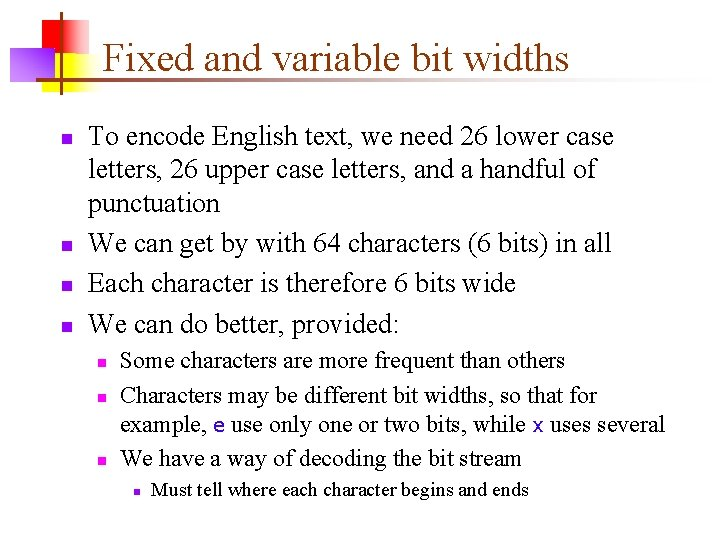Fixed and variable bit widths n n To encode English text, we need 26 lower case letters, 26 upper case letters, and a handful of punctuation We can get by with 64 characters (6 bits) in all Each character is therefore 6 bits wide We can do better, provided: n n n Some characters are more frequent than others Characters may be different bit widths, so that for example, e use only one or two bits, while x uses several We have a way of decoding the bit stream n Must tell where each character begins and ends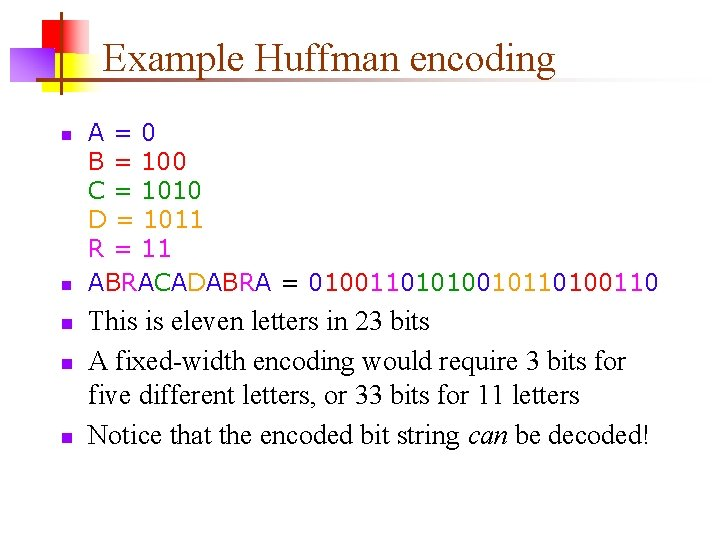Example Huffman encoding n n n A=0 B = 100 C = 1010 D = 1011 R = 11 ABRACADABRA = 01001101010010110100110 This is eleven letters in 23 bits A fixed-width encoding would require 3 bits for five different letters, or 33 bits for 11 letters Notice that the encoded bit string can be decoded!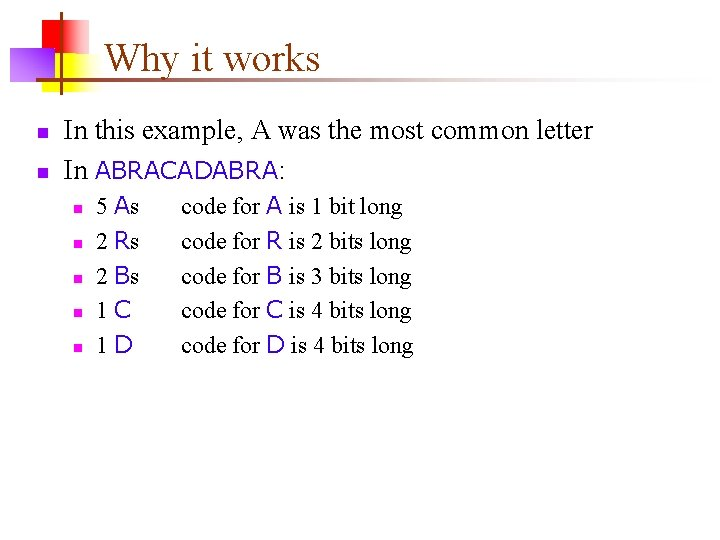Why it works n n In this example, A was the most common letter In ABRACADABRA: n n n 5 As 2 Rs 2 Bs 1 C 1 D code for A is 1 bit long code for R is 2 bits long code for B is 3 bits long code for C is 4 bits long code for D is 4 bits long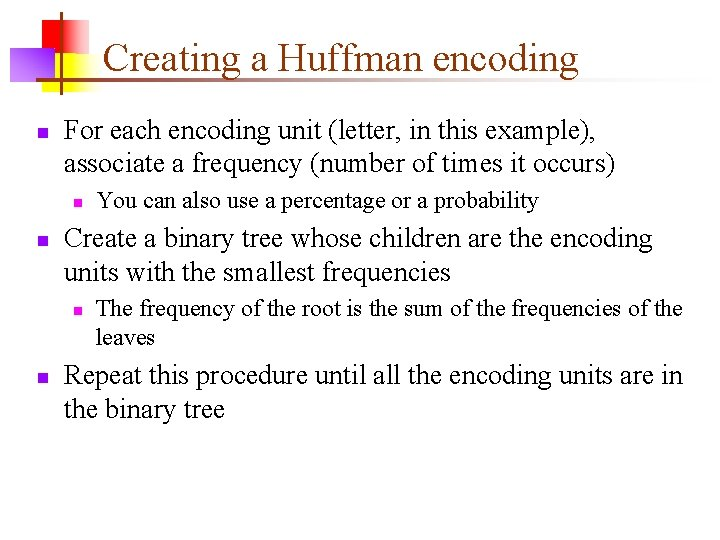Creating a Huffman encoding n For each encoding unit (letter, in this example), associate a frequency (number of times it occurs) n n Create a binary tree whose children are the encoding units with the smallest frequencies n n You can also use a percentage or a probability The frequency of the root is the sum of the frequencies of the leaves Repeat this procedure until all the encoding units are in the binary tree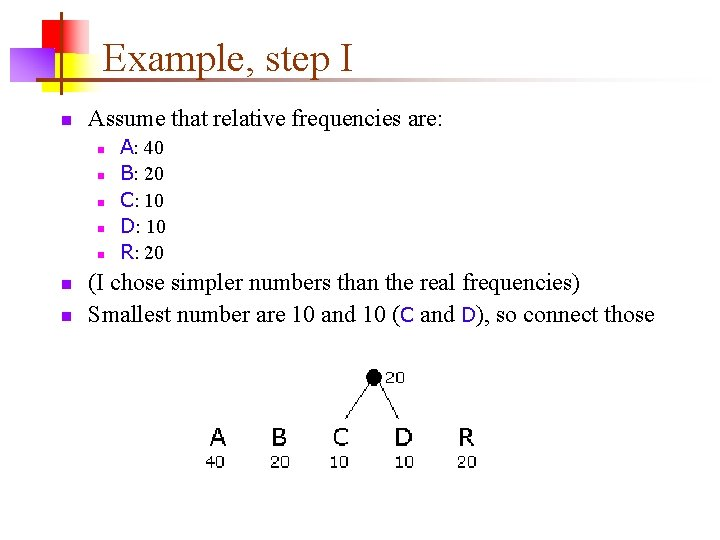Example, step I n Assume that relative frequencies are: n n n n A: 40 B: 20 C: 10 D: 10 R: 20 (I chose simpler numbers than the real frequencies) Smallest number are 10 and 10 (C and D), so connect those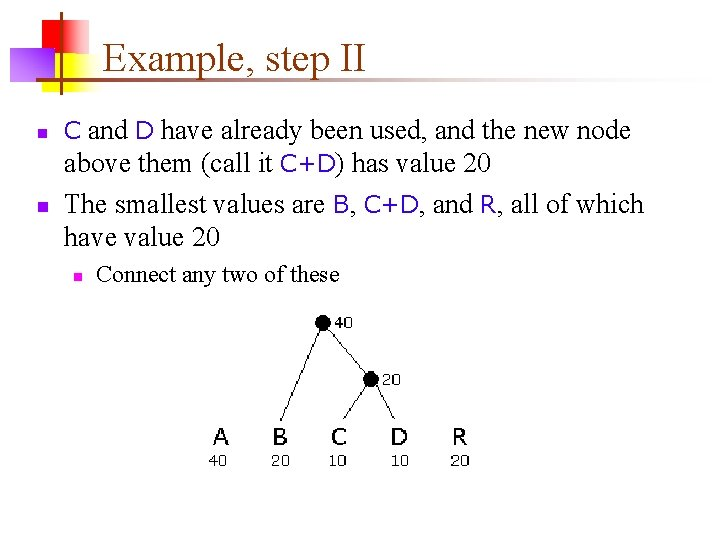Example, step II n n C and D have already been used, and the new node above them (call it C+D) has value 20 The smallest values are B, C+D, and R, all of which have value 20 n Connect any two of these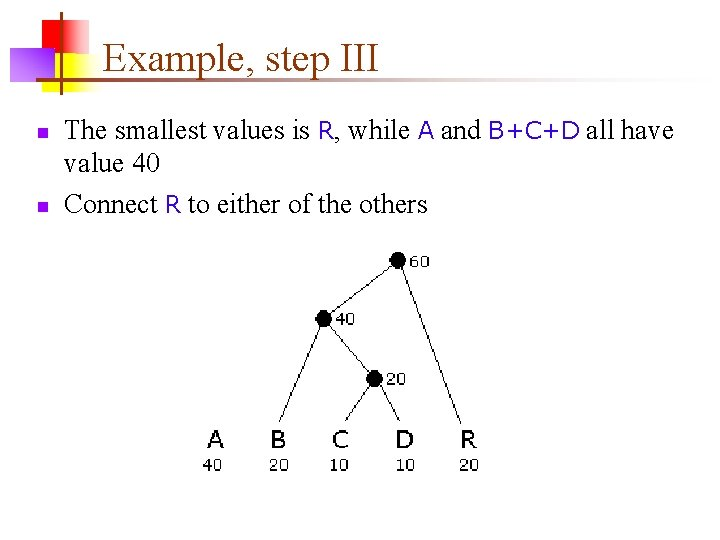Example, step III n n The smallest values is R, while A and B+C+D all have value 40 Connect R to either of the others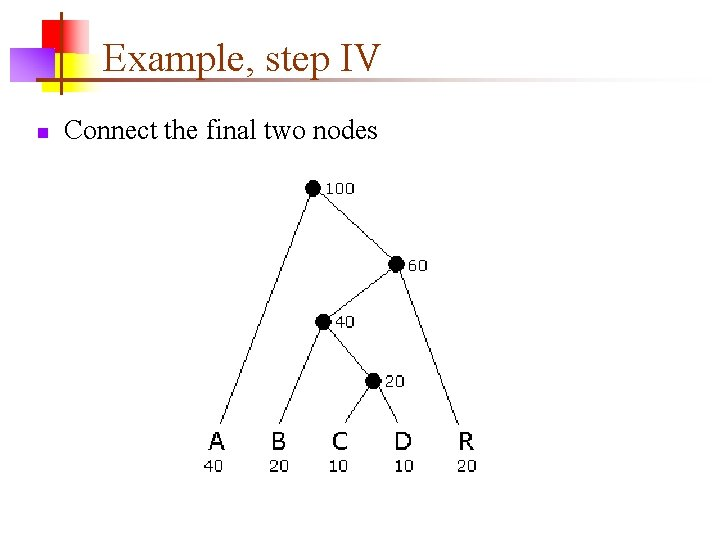Example, step IV n Connect the final two nodesExample, step V n n Assign 0 to left branches, 1 to right branches Each encoding is a path from the root n n n A=0 B = 100 C = 1010 D = 1011 R = 11 Each path terminates at a leaf Do you see why encoded strings are decodable?Unique prefix property n n n A=0 B = 100 C = 1010 D = 1011 R = 11 No bit string is a prefix of any other bit string For example, if we added E=01, then A (0) would be a prefix of E Similarly, if we added F=10, then it would be a prefix of three other encodings (B=100, C=1010, and D=1011) The unique prefix property holds because, in a binary tree, a leaf is not on a path to any other node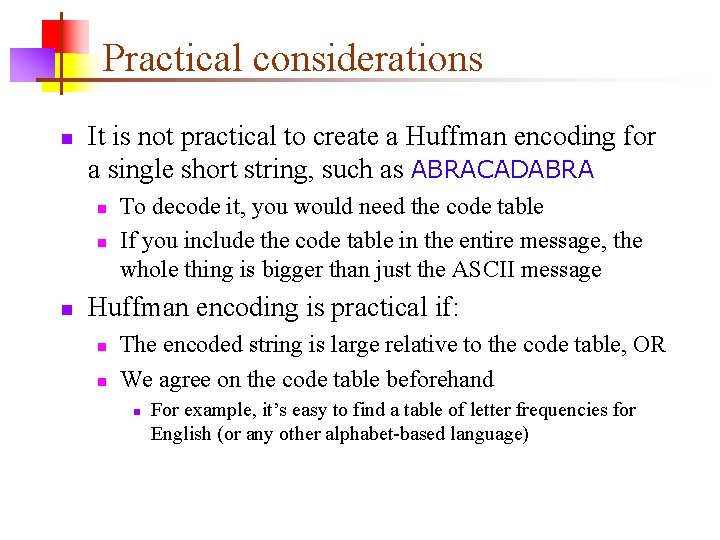Practical considerations n It is not practical to create a Huffman encoding for a single short string, such as ABRACADABRA n n n To decode it, you would need the code table If you include the code table in the entire message, the whole thing is bigger than just the ASCII message Huffman encoding is practical if: n n The encoded string is large relative to the code table, OR We agree on the code table beforehand n For example, it’s easy to find a table of letter frequencies for English (or any other alphabet-based language)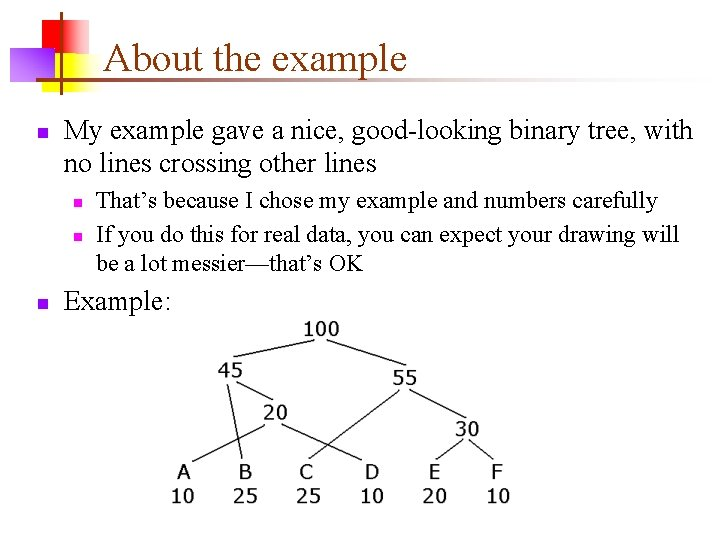About the example n My example gave a nice, good-looking binary tree, with no lines crossing other lines n n n That’s because I chose my example and numbers carefully If you do this for real data, you can expect your drawing will be a lot messier—that’s OK Example:Data compression n Huffman encoding is a simple example of data compression: representing data in fewer bits than it would otherwise need A more sophisticated method is GIF (Graphics Interchange Format) compression, for. gif files Another is JPEG (Joint Photographic Experts Group), for. jpg files n n Unlike the others, JPEG is lossy—it loses information Generally OK for photographs (if you don’t compress them too much), because decompression adds “fake” data very similiar to the original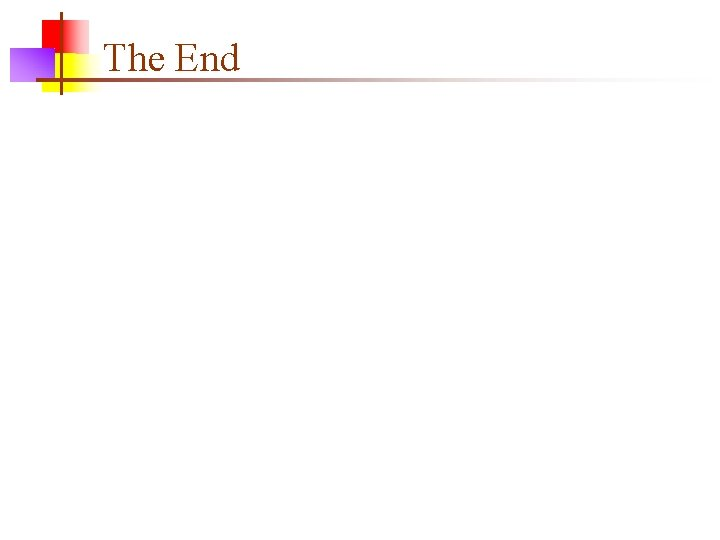The End